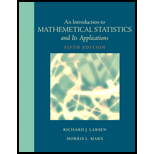# An Introduction to Mathematical Statistics and Its Applications5th Edition### Sorry! We don’t have solutions for this edition yet.

#### Solutions are available for other editions of this book

View 6th Edition solutionsarrow_forward

### Book Details

1. Introduction

1.1 An Overview

1.2 Some Examples

1.3 A Brief History

1.4 A Chapter Summary

2. Probability

2.1 Introduction

2.2 Sample Spaces and the Algebra of Sets

2.3 The Probability Function

2.4 Conditional Probability

2.5 Independence

2.6 Combinatorics

2.7 Combinatorial Probability

2.8 Taking a Second Look at Statistics (Monte Carlo Techniques)

3. Random Variables

3.1 Introduction

3.2 Binomial and Hypergeometric Probabilities

3.3 Discrete Random Variables

3.4 Continuous Random Variables

3.5 Expected Values

3.6 The Variance

3.7 Joint Densities

3.8 Transforming and Combining Random Variables

3.9 Further Properties of the Mean and Variance

3.10 Order Statistics

3.11 Conditional Densities

3.12 Moment-Generating Functions

3.13 Taking a Second Look at Statistics (Interpreting Means)

Appendix 3.A.1 MINITAB Applications

4. Special Distributions

4.1 Introduction

4.2 The Poisson Distribution

4.3 The Normal Distribution

4.4 The Geometric Distribution

4.5 The Negative Binomial Distribution

4.6 The Gamma Distribution

4.7 Taking a Second Look at Statistics (Monte Carlo Simulations)

Appendix 4.A.1 MINITAB Applications

Appendix 4.A.2 A Proof of the Central Limit Theorem

5. Estimation

5.1 Introduction

5.2 Estimating Parameters: The Method of Maximum Likelihood and the Method of Moments

5.3 Interval Estimation

5.4 Properties of Estimators

5.5 Minimum-Variance Estimators: The Cramér-Rao Lower Bound

5.6 Sufficient Estimators

5.7 Consistency

5.8 Bayesian Estimation

5.9 Taking A Second Look at Statistics (Beyond Classical Estimation)

Appendix 5.A.1 MINITAB Applications

6. Hypothesis Testing

6.1 Introduction

6.2 The Decision Rule

6.3 Testing Binomial Data–H0: p = po

6.4 Type I and Type II Errors

6.5 A Notion of Optimality: The Generalized Likelihood Ratio

6.6 Taking a Second Look at Statistics (Statistical Significance versus “Practical” Significance)

7. Inferences Based on the Normal Distribution

7.1 Introduction

7.2 Comparing Y-? ?/ ?n and Y-? S/ ?n

7.3 Deriving the Distribution of Y-? S/ ?n

7.6 Taking a Second Look at Statistics (Type II Error)

Appendix 7.A.1 MINITAB Applications

Appendix 7.A.2 Some Distribution Results for Y and S2

Appendix 7.A.3 A Proof that the One-Sample t Test is a GLRT

Appendix 7.A.4 A Proof of Theorem 7.5.2

8. Types of Data: A Brief Overview

8.1 Introduction

8.2 Classifying Data

8.3 Taking a Second Look at Statistics (Samples Are Not “Valid”!)

9. Two-Sample Inferences

9.1 Introduction

9.2 Testing H0: ?X =?Y

9.3 Testing H0: ?2X=?2Y–The F Test

9.4 Binomial Data: Testing H0: pX = pY

9.5 Confidence Intervals for the Two-Sample Problem

9.6 Taking a Second Look at Statistics (Choosing Samples)

Appendix 9.A.1 A Derivation of the Two-Sample t Test (A Proof of Theorem 9.2.2)

Appendix 9.A.2 MINITAB Applications

10. Goodness-of-Fit Tests

10.1 Introduction

10.2 The Multinomial Distribution

10.3 Goodness-of-Fit Tests: All Parameters Known

10.4 Goodness-of-Fit Tests: Parameters Unknown

10.5 Contingency Tables

10.6 Taking a Second Look at Statistics (Outliers)

Appendix 10.A.1 MINITAB Applications

11. Regression

11.1 Introduction

11.2 The Method of Least Squares

11.3 The Linear Model

11.4 Covariance and Correlation

11.5 The Bivariate Normal Distribution

11.6 Taking a Second Look at Statistics (How Not to Interpret the Sample Correlation Coefficient)

Appendix 11.A.1 MINITAB Applications

Appendix 11.A.2 A Proof of Theorem 11.3.3

12. The Analysis of Variance

12.1 Introduction

12.2 The F Test

12.3 Multiple Comparisons: Tukey’s Method

12.4 Testing Subhypotheses with Contrasts

12.5 Data Transformations

12.6 Taking a Second Look at Statistics (Putting the Subject of Statistics together–the Contributions of Ronald A. Fisher)

Appendix 12.A.1 MINITAB Applications

Appendix 12.A.2 A Proof of Theorem 12.2.2

Appendix 12.A.3 The Distribution of SSTR/(k—1) SSE/(n—k)When H1 is True

13. Randomized Block Designs

13.1 Introduction

13.2 The F Test for a Randomized Block Design

13.3 The Paired t Test

13.4 Taking a Second Look at Statistics (Choosing between a Two-Sample t Test and a Paired t Test)

Appendix 13.A.1 MINITAB Applications

14. Nonparametric Statistics

14.1 Introduction

14.2 The Sign Test

14.3 Wilcoxon Tests

14.4 The Kruskal-Wallis Test

14.5 The Friedman Test

14.6 Testing for Randomness

14.7 Taking a Second Look at Statistics (Comparing Parametric and Nonparametric Procedures)

Appendix 14.A.1 MINITAB Applications

Appendix: Statistical Tables